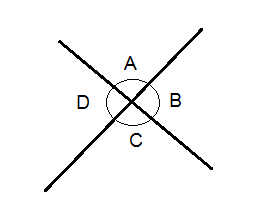# Angle facts

?
• Created by: holly6901
• Created on: 25-05-19 13:51

## Opposite angles

Opposite angles are equal1 of 10

## Angles on a straight lineAngles on a straight line add up to 180

2 of 103 of 10

## Alternate anglesAlternate angles are equal to each other

4 of 105 of 10

## Angles in a polygonThe interior angles in a polygon with n sides add up to (n-2)x180

6 of 10

## Angles in a right angle triangleIn a right angle triangle, the other two angles are equal to 90

7 of 10

## Angles in an equilateral triangleIn an equilateral triangle, all angles are 60

8 of 10

## Corresponding anglesCorresponding angles are equal, and make an F shape

9 of 10

## Co-interior angles